Disclosure: This post may contain affiliate links, meaning when you click the links and make a purchase, we receive a commission.

# What is Spreadsheet in MS Excel

A spreadsheet in MS Excel is basically a worksheet which is divided into rows and columns to store data related to business inventories, income, and expenses, debits and credits. Today’s business world uses electronic spreadsheets in place of antiquated paper-based worksheets.

You can change the value stored in a spreadsheet cell. Changing the value in old-fashioned paper-based worksheet was toilsome as it created tedious manual recalculation.

An MS Excel spreadsheet is made up of three things-rows, columns, and their intersections, cells. Columns are typically labeled using letters (A, B, C, D, …) and rows are typically labeled using numbers (1, 2, 3, 4, …). An intersection of a column and a row makes a cell in an MS Excel spreadsheet.

### What is an MS Excel Spreadsheet Column

In an MS Excel spreadsheet, the column is the vertical space that is going up and down the spreadsheet. The highlighted part of the following spreadsheet is a column and it is labeled B.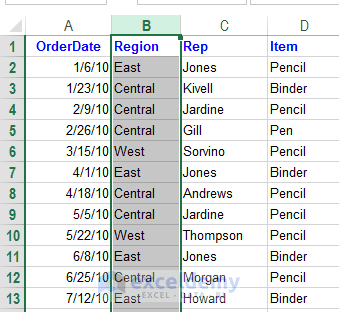An MS Excel Spreadsheet column. The highlighted part is column B.

### What is an MS Excel Spreadsheet Row

In an MS Excel spreadsheet, the row is the horizontal space that is going across the spreadsheet. The highlighted part of the following spreadsheet is a row and it is labeled number 5.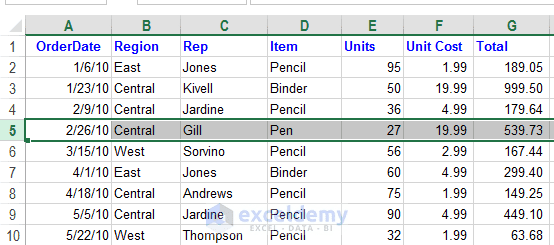Spreadsheet Row. The highlighted part is row 5.

### What is MS Excel Spreadsheet Cell

In an MS Excel spreadsheet, a cell is a place where a column and a row intersect. Every cell is assigned an address with its column letter and row number. Remember column letter will come first and row number will be placed second in a cell’s address. An Excel 2013 spreadsheet is made up of more than 17 billion cells.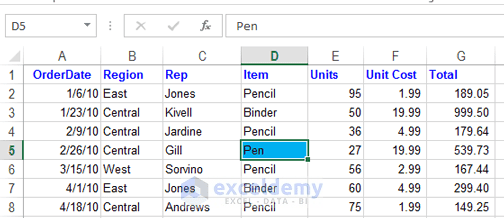Spreadsheet Cell. Highlighted space is a cell created by the cross-section of column D and row 5, cell address D5.

### Last Cell in an MS Excel Spreadsheet

In an Excel 2013 spreadsheet, column letters range from A to XFD, total 16,384 columns and row numbers range from 1 to 10, 48,576. The last cell address is then XFD1048576 in an Excel spreadsheet.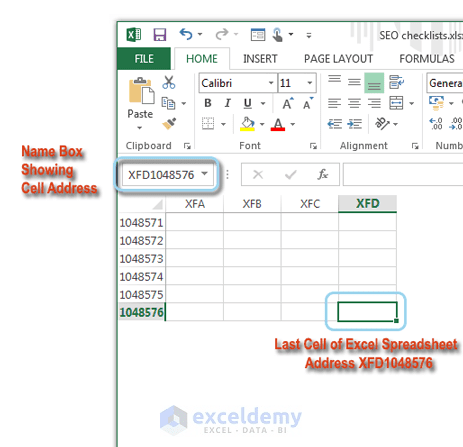Last cell of an Excel 2013 spreadsheet.

### What is MS Excel Spreadsheet-the Data Types you can store in the Cells

A modern spreadsheet is made up of a huge number of cells. A Microsoft’s Excel 2013 spreadsheet holds more than 17 billion cells. A cell holds three basic types of data:

• A numeric value:
A numeric value can be a general number, a scientific number, date, time, or a formatted number. Dates (such as Feb-26-2013) or times (such as 3:24 a.m.) are considered as numeric values.
• Text:
Texts can be labels for values, headings for columns, or instructions about a spreadsheet. Text if begins with numbers or text with numbers is still considered text. For example ‘6/1 Block C’ is text ‘as well as Block C, 6/1’.
• A formula:
Formulas are what make a spreadsheet a spreadsheet. When you enter a formula into a cell, the formula’s result appears in the cell. Formulas can be simple mathematical expressions, or they can use some of the powerful functions that are built into Excel.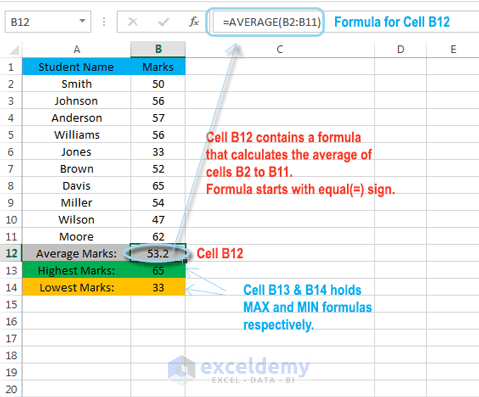What is spreadsheet cell can contain

### What is MS Excel Spreadsheet Invisible Draw Layer

An MS Excel spreadsheet can also hold charts, diagrams, pictures, buttons, and other objects. But these objects aren’t contained in cells. Instead, they reside on the worksheet’s draw layer, which is an invisible layer on top of each worksheet.

Excel is the most used spreadsheet program in the world and industry standard. There are other Excel programs but Excel is the most popular.

Hello!
Welcome to my Excel blog! It took me some time to be a fan of Excel. But now I am a die-hard fan of MS Excel. I learn new ways of doing things with Excel and share here. Not only how to guide on Excel, but you will get also topics on Finance, Statistics, Data Analysis, and BI. Stay tuned!

We will be happy to hear your thoughts